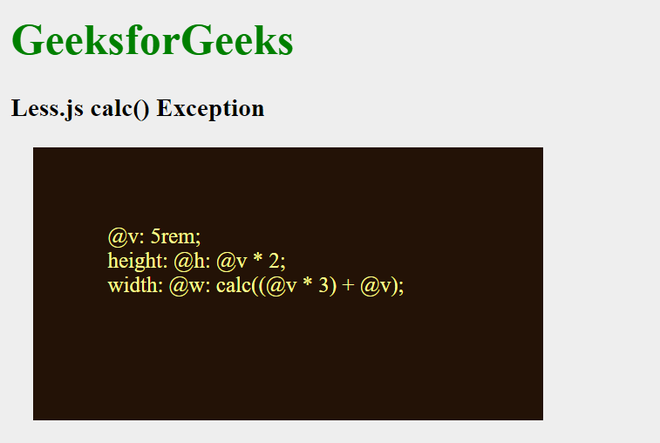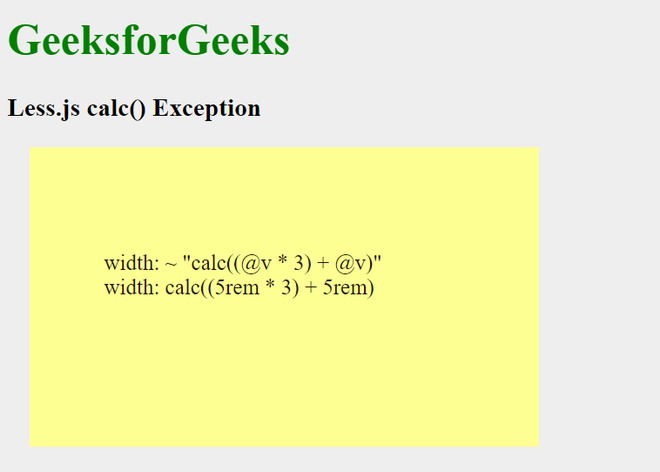GFG App
Open AppBrowser
Continue

Less.js is a simple CSS pre-processor that facilitates the creation of manageable, customizable, and reusable style sheets for websites. Since CSS is a dynamic style sheet language, it is preferred. Because LESS is adaptable, it can be used by a variety of browsers. Only CSS that has been created and processed using the CSS pre-processor, a computer language, can be used by web browsers. In addition to providing capabilities like variables, functions, mixins, and operations to help create dynamic CSS while maintaining backward compatibility, it is a CSS language extension.

The built-in CSS function calc() is used to carry out computations based on CSS properties. The CSS calc() method performs calculations using either the values of its parent element or the values entered by the user.

For more CSS compatibility, in less.js the calc function is disabled for evaluating the math expressions and when they are described inside nested functions are evaluated. But if we want the calc function to not change anything and keep it as it is then we can add the expression inside “” quotes and put a ~ before it so that after compilation, the expression will be the same as it is.

Syntax:

`calc(expression)`

Parameters:

• expression: The is a compulsory parameter which is the main Math expression.

Compile LESS code into CSS code.

Example 1: The example below practically illustrates the exception in the calc() function and how the nested functions are solved.

## HTML

 ` ` `<``html``> ` ` `  `<``head``> ` `    ``<``link` `rel``=``"stylesheet"` `type``=``"text/css"`  `          ``href``=``"style.css"``/> ` ` ` ` `  `<``body``> ` `    ``<``h1` `style``=``"color:green"``>GeeksforGeeks   ` `    ``<``h3``><``b``>Less.js calc() Exception ` `    ``<``div` `class``=``"c1"``>   ` `        ``<``p``>@v: 5rem;<``br``> ` `        ``height: @h: @v * 2;<``br``> ` `        ``width: @w: calc((@v * 3) + @v);  ` `    `` ` ` ` ` `  ``

styles.less

## CSS

 `@body-bg-``color``: ``#eeeeee``; ` `@dark: hsl(``25``, ``71%``, ``8%``); ` `@light: ``rgb``(``253``, ``255``, ``146``); ` `@v: ``5``rem; ` `@h: @v * ``2``; ` `@w: calc((@v * ``3``) + @v); ` `body { ` `    ``background-color``: @body-bg-color; ` `} ` `.c``1` `{ ` `    ``width``: @w; ` `    ``height``: @h; ` `    ``margin``: ``1``rem; ` `    ``background-color``: @dark; ` `    ``padding``: ``40px` `0px` `0px` `55px``; ` `} ` `p { ` `    ``color``: @light; ` `}`

Now, to compile the above LESS code to CSS code, run the following command:

`lessc styles.less styles.css`

The compiled CSS file comes to be:

style.css: The CSS output of the above Less file was compiled.

## CSS

 `body { ` `    ``background-color``: ``#eeeeee``; ` `} ` `.c``1` `{ ` `    ``width``: calc((``5``rem * ``3``) + ``5``rem); ` `    ``height``: ``10``rem; ` `    ``margin``: ``1``rem; ` `    ``background-color``: hsl(``25``, ``71%``, ``8%``); ` `    ``padding``: ``40px` `0px` `0px` `55px``; ` `} ` `p { ` `    ``color``: ``#fdff92``; ` `}`

Output:Example 2: The example below practically illustrates the exception in calc() function and how using the ~ with string form will let is be as it is.

## HTML

 ` ` `<``html``> ` ` `  `<``head``> ` `    ``<``link` `rel``=``"stylesheet"` `type``=``"text/css"` `          ``href``=``"style.css"``/> ` ` ` ` `  `<``body``> ` `    ``<``h1` `style``=``"color:green"``>GeeksforGeeks   ` `    ``<``h3``><``b``>Less.js calc() Exception ` `    ``<``div` `class``=``"c1"``>   ` `        ``<``p``>width: ~ "calc((@v * 3) + @v)"<``br``> ` `            ``width: calc((5rem * 3) + 5rem)  ` `    `` ` ` ` ` `  ``

styles.less

## CSS

 `@body-bg-``color``: ``#eeeeee``; ` `@dark: hsl(``25``, ``71%``, ``8%``); ` `@light: ``rgb``(``253``, ``255``, ``146``); ` `@v: ``5``rem; ` `@h: @v * ``2``; ` `body { ` `    ``background-color``: @body-bg-color; ` `} ` `.c``1` `{ ` `    ``width``: ~ ``"calc((5rem * 3) + 5rem)"``; ` `    ``height``: @h; ` `    ``margin``: ``1``rem; ` `    ``background-color``: @light; ` `    ``padding``: ``60px` `0px` `0px` `55px``; ` `} ` `p { ` `    ``color``: @dark; ` `}`

Now, to compile the above LESS code to CSS code, run the following command:

`lessc styles.less styles.css`

The compiled CSS file comes to be:

style.css: The CSS output of the above Less file was compiled.

## CSS

 `body { ` `    ``background-color``: ``#eeeeee``; ` `} ` `.c``1` `{ ` `    ``width``: calc((``5``rem * ``3``) + ``5``rem); ` `    ``height``: ``10``rem; ` `    ``margin``: ``1``rem; ` `    ``background-color``: ``#fdff92``; ` `    ``padding``: ``60px` `0px` `0px` `55px``; ` `} ` `p { ` `    ``color``: hsl(``25``, ``71%``, ``8%``); ` `}`

Output:My Personal Notes arrow_drop_up